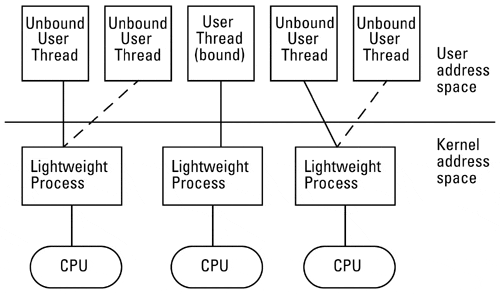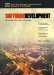There are at least two ways to take advantage of more than one CPU in a multiprocessor system. The first way is to run multiple applications, or multiple copies of the same application. Often, however, one desires to utilize a multiprocessor system to increase the performance of a single application, such as a DBMS or a large scientific program. To do so requires that the program itself be multithreaded, allowing it to be broken up into multiple sequences of instructions that can execute in parallel.

A software developer has three basic options to create a multithreaded program. First, an automatic parallelizing compiler can be used with existing non-threaded source code. Automatic parallelizing works best with simply structured languages such as FORTRAN and with scientific code. Scientific code tends to have large numbers of loops with many iterations and no data dependencies. Such code can be easily multithreaded by a compiler. For instance, consider the code segment:

` void makeSums() {    int i;    double a, b, c;    double sum1, sum2;    /* initialize a, b, and c arrays */        for (i = 0; i < 100; i++){       sum1[i] = a[i] + b[i];       sum2[i] = b[i] + c[i];    } } `

Assuming each addition takes one time unit to execute, the above code could execute in 200 time units. Since there are no data dependencies in this code, it could be re-written by a compiler into two sub-functions or threads that could execute independently as follows :

` /* global variables */ double a, b, c; double sum1, sum2; void makeSums() {     /* initialize a, b, and c arrays */          thread_create(thread1);     thread_create(thread2); } void thread1()    int i;    for (i = 0; i < 100; i++){       sum1[i] = a[i] + b[i];    } } /* Function thread2 */ void thread2() {    int i;    for (i = 0; i < 100; i++){       sum2[i] = b[i] + c[i];    } } `

Assume there are two CPUs available to execute the code. The program will now execute in 100 time units, plus any overhead needed to start up the threads.

The second way a developer can create multithreaded code is to insert compiler directives into the source code, manually identifying which loops and other sections of code are candidates for multithreading. This can be useful if the code is structured such that the compiler cannot automatically parallelize the code.

The third method, which generally has the highest performance payback, is to design multithreaded code. As with many of the concepts presented in this book, multithreading has the greatest advantage if it is considered early in the design stage versus trying to retrofit threads into a non-concurrent program.

Two important concepts to understand when discussing multithreading code are those of concurrency and parallelism. Concurrency exists when at least two threads are in progress at the same time. This may occur even in a single CPU system. For instance, one thread might be accepting input from the user interface while another thread is updating the database and waiting for a response from the database server. Because of concurrency, multithreaded code often executes faster even on single CPU systems. Parallelism arises when at least two threads are executing at the same time on a multi-CPU system. On a multi-CPU system, a multithreaded program can take advantage of both concurrency and multithreading.

##### Figure 19-1. Posix Two-Level Threads Model##### Table19-1. Thread Creation Times, Solaris 2.6, 200 MHz Ultra 1
Operation Time (Milliseconds) Ratio
Create bound thread and new LWP 400 4.0
Create new process with fork() 5000 50Software Development: Building Reliable Systems
ISBN: 0130812463
EAN: 2147483647
Year: 1998
Pages: 193
Authors: Marc Hamilton